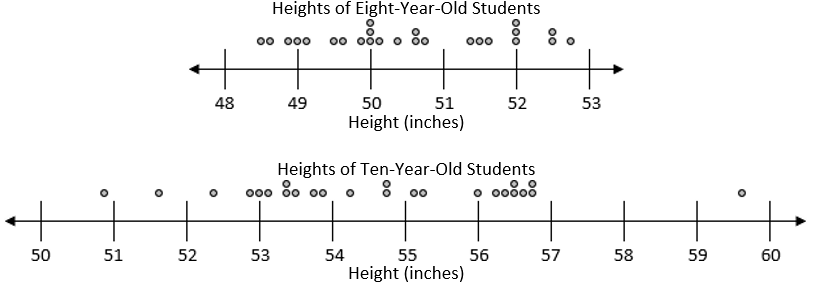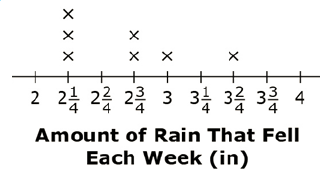Match Fishtank is now Fishtank Learning!

# Fraction Operations

## Objective

Solve problems by using information presented in line plots.

## Common Core Standards

### Core Standards

?

• 4.MD.B.4 — Make a line plot to display a data set of measurements in fractions of a unit (1/2, 1/4, 1/8). Solve problems involving addition and subtraction of fractions by using information presented in line plots. For example, from a line plot find and interpret the difference in length between the longest and shortest specimens in an insect collection.

?

• 3.MD.B.4

## Criteria for Success

?

1. Solve word problems involving information presented in a line plot (MP.4), including questions that ask students to:
1. Find the sum of values (or a subset of values) presented in the line plot and
2. Find the difference between the largest and smallest values in the line plot.
2. Construct a line plot (as a spiral from Lesson 21).
3. Reason inductively about data in a line plot to make a plausible argument that takes into account the context from which the data arose (e.g., based on this data, what is typical?) (MP.3).

## Tips for Teachers

?

“Grade 4 students learn elements of fraction equivalence (4.NF.1) and arithmetic, including multiplying a fraction by a whole number (4.NF.4) and adding and subtracting fractions with like denominators (4.NF.3). Students can use these skills to solve problems, including problems that arise from line plots” (MD Progression, p. 11).

#### Remote Learning Guidance

If you need to adapt or shorten this lesson for remote learning, we suggest prioritizing Anchor Task 1 (benefits from worked example). Find more guidance on adapting our math curriculum for remote learning here.

#### Fishtank Plus

• Problem Set
• Student Handout Editor
• Vocabulary Package

?

### Problem 1

Look back at yesterday’s data regarding the distances Ms. Smith’s class ran.

1. How many students ran less than ${2{1\over2}}$ miles?
2. What is the difference between the longest and the shortest distance ran?
3. What is the total distance the students ran?

#### References

EngageNY Mathematics Grade 4 Mathematics > Module 5 > Topic E > Lesson 28Concept Development

Grade 4 Mathematics > Module 5 > Topic E > Lesson 28 of the New York State Common Core Mathematics Curriculum from EngageNY and Great Minds. © 2015 Great Minds. Licensed by EngageNY of the New York State Education Department under the CC BY-NC-SA 3.0 US license. Accessed Dec. 2, 2016, 5:15 p.m..

Modified by Fishtank Learning, Inc.

### Problem 2

Fourth-grade students interested in seeing how heights of students change for kids around their age measured the heights of a sample of eight-year-olds and a sample of ten-year-olds. Their data are plotted below.Describe the key differences between the heights of these two age groups.

#### References

Institute for Mathematics and Education Progression for the Common Core State Standards, K–3, Categorical Data; Grades 2–5, Measurement Datap. 13

Modified by Fishtank Learning, Inc.

## Problem Set & Homework

#### Discussion of Problem Set

• Which expression is correct in #1? How do you know?
• In #2, how can you tell that options (c) and (d) are unreasonable? How might estimation help you?
• How did multiplication help you with certain computations? Which problems did you use multiplication to help solve?

?

A student measured how much rain fell each week. This line plot shows the amount of rain, in inches, that fell each week.1. How much more rain, in inches, was there during the week with the greatest amount of rain than the week with the least amount of rain?
2. How much did it rain in total?

### Mastery Response

?